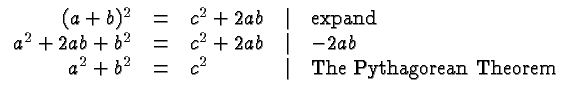# Mathematics 1010 online

## The Pythagorean Theorem

Some of the homework problems in this class require the Pythagorean Theorem, named after Pythagoras who lived approximately 380-300 BC. That Theorem is also used for example when computing the distance between two points in the Cartesian Coordinate System.

The Pythagorean Theorem states that in a right triangle the squares of the two short sides add to the square of the long side. If we call the lengths of the two short sidesand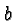, and the length of the long side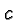this leads to the familiar statement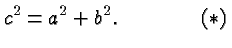Of course, the sides need not be called,and, and the reverse of the above statement also holds: if the equation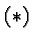holds than the triangle in question is a right triangle.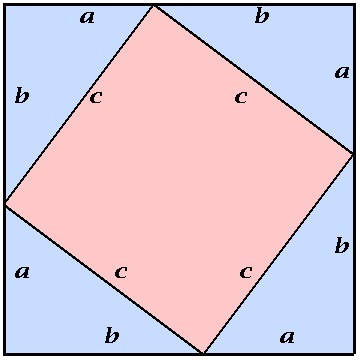The Pythagorean Theorem

The Figure on this page illustrates a simple proof of the Pythagorean Theorem. We describe that proof here because it provides a beautiful application of Intermediate Algebra. Take four right triangles (shown in blue) with sides,andand line them up as indicated in the Figure. Thus we continue the sideof one triangle with the sideof another. The result is a square whose sides have length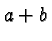. It encloses a smaller square (shown in red) whose sides have length.

Let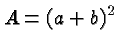denote the area of the large square. It can also be computed by adding the areas of the four triangles and the area of the inner square. The area of one blue triangle equals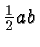(which is half of base times height). Thus we also have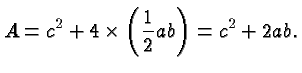The two expressions are equal and we obtain: